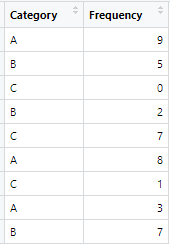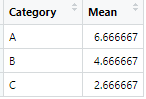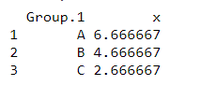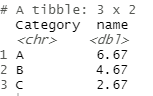Related Articles

# How to Calculate the Mean by Group in R DataFrame ?

• Last Updated : 01 Apr, 2021

In this article, we are going to see how to calculate the mean by the group in DataFrame in R Programming Language.

It can be done with two approaches:

Dataset creation: First, we create a dataset so that later we can apply the above two approaches and find the Mean by group.

## R

 `# GFG dataset name and creation``GFG <- ``data.frame``(                                            ``   ``Category  = ``c ``(``"A"``,``"B"``,``"C"``,``"B"``,``"C"``,``"A"``,``"C"``,``"A"``,``"B"``),       ``   ``Frequency= ``c``(9,5,0,2,7,8,1,3,7)                            ``)`` ` `# Prints the dataset``print``(GFG)                                                    `

So, as you can see the above code is for creating a dataset named “GFG”.

It also has 2 columns named Category and Frequency. So, when you run the above code in an R compiler, a table is shown as output as given belowAnd after applying that two approaches we need to get output as:Before we discuss those approaches let us first know how we got the output values:

• In Table 1, We have two columns named Category and Frequency.
• In Category, we have some repeating variables of A, B and C.
• A group values (9,8,3), B group values (5,2,7) and C group values (0,7,1) taken from the Frequency column.
• So, to find Mean we have a  formula

MEAN = Sum of terms / Number of terms

• Hence, Mean by Group  of each group (A,B,C) would be

Sum:

• A=9+8+3=20
• B=5+2+7=14
• C=0+7+1=08

Number of terms:

• A is repeated 3 times
• B is repeated 3 times
• C is repeated 3 times

Mean by group (A, B, C):

• A(mean) = Sum/Number of terms = 20/3 = 6.67
• B(mean) = Sum/Number of terms = 14/3 = 4.67
• C(mean) = Sum/Number of terms = 8/3 = 2.67

Method 1: Using aggregate function

Aggregate function: Splits the data into subsets, computes summary statistics for each, and returns the result in a convenient form.

Syntax: aggregate(x = dataset_Name , by = group_list, FUN = any_function)

# Basic R syntax of aggregate function

Now, let’s sum our data using an aggregate function:

## R

 `GFG <- ``data.frame``(``   ``Category  = ``c ``(``"A"``,``"B"``,``"C"``,``"B"``,``"C"``,``"A"``,``"C"``,``"A"``,``"B"``), ``   ``Frequency= ``c``(9,5,0,2,7,8,1,3,7)``)`` ` `# Specify data column``aggregate``(x= GFG\$Frequency,     ``           ` `         ``# Specify group indicator``         ``by = ``list``(GFG\$Category),      ``           ` `         ``# Specify function (i.e. mean)``         ``FUN = mean)`

Output:In the above aggregate function, it takes on three parameters

• First is dataset name in our case it is “GFG”.
• Second is the column name which values we need to make different groups in our case it is Category column, and it is separated into three groups (A, B, C).
• In the third parameter, we need to mention which function(i.e mean, sum, etc) we need to perform on a group formed (A, B, C)

Method 2: Using dplyr Package

dplyr is a package which provides a set of tools for efficiently manipulating datasets in R

Methods in dplyr package:

• mutate() adds new variables that are functions of existing variables
• select() picks variables based on their names.
• filter() picks cases based on their values.
• summarise() reduces multiple values down to a single summary.
• arrange() changes the ordering of the rows.

Install this library:

`install.packages("dplyr") `

`library("dplyr")  `

Code:

## R

 `# load dplyr library``library``(``"dplyr"``)                             ``GFG <- ``data.frame``(``   ``Category  = ``c ``(``"A"``,``"B"``,``"C"``,``"B"``,``"C"``,``"A"``,``"C"``,``"A"``,``"B"``), ``   ``Frequency= ``c``(9,5,0,2,7,8,1,3,7)``)`` ` `# Specify data frame``GFG%>%                                        `` ` `# Specify group indicator, column, function``group_by``(Category) %>%                        ``summarise_at``(``vars``(Frequency),``              ``list``(name = mean))`

Output:In the above code, we first take our dataset named “GFG”. With group_by() method we form groups in our case (A, B, C). summarise_at() it has two parameters first is a column on which it applies the operation given as the second parameter of it.

My Personal Notes arrow_drop_up